A6.2 Reinforcement Learning to Control a Robot

• A6.2: Modified the incomplete code for the train function. You can ignore this version if you have already achieved successful training of your robot.

• A6.1: To help you test your code, I added a call to np.random.seed to allow you to compare your output with mine. At start of Section 4, I added a statement saying that you must discuss what you observe in the results. Also added an argument to the Robot.animate function to allow you to draw one plot to visualize the trained robot's behavior.

In this assignment, you will play with a simulation of a two-dimensional robot arm with multiple links and joints. You will train a neural network to approximate the Q function.

The state of the arm is just the angles of each joint. To remove the huge difference between joint angles of 359 degrees and 1 degree as a joint moves, we will represent each joint angle with two values, the sine and cosine of the angle, as the state input to the neural network. Valid actions on each step will be $-0.1$, $0$, and $+0.1$ applied to each joint.

# Robot class¶

Here is an implementation of the robot simulation.

In :
import numpy as np
import copy
import matplotlib.pyplot as plt
import sys
import itertools  # for product (cross product)
from math import pi
from IPython.display import display, clear_output


Now import your NeuralNetwork regression class that uses numpy and has the scg optimizer available.

In :
import A3mysolution as nn  # where you implement the NeuralNetwork class

In :
class Robot():

self.record_angles = False
self.angles = []
self.points = [[10, 10] for _ in range(self.n_links + 1)]
self.update_points()
self.goal = None

def set_goal(self, g):
self.goal = g

def dist_to_goal(self):
return np.sqrt(np.sum((self.goal - self.end_effector)**2))

def update_joints(self, joint_angles):
self.joint_angles = joint_angles
self.update_points()

self.joint_angles += joint_angle_deltas
too_high = self.joint_angles > 2 * pi
self.joint_angles[too_high] = self.joint_angles[too_high] - 2 * pi
too_low = self.joint_angles < 0
self.joint_angles[too_low] = self.joint_angles[too_low] + 2 * pi
if self.record_angles:
self.angles.append(self.joint_angles * 180 / pi)
self.update_points()

def update_points(self):
for i in range(1, self.n_links + 1):
self.points[i] = (self.points[i - 1]
+ self.link_lengths[i - 1] * np.cos(np.sum(self.joint_angles[:i])))
self.points[i] = (self.points[i - 1] +

def get_angles(self):
return self.joint_angles

def plot(self, style):
for i in range(self.n_links + 1):
plt.plot([self.points[i], self.points[i + 1]],
[self.points[i], self.points[i + 1]], style)
plt.plot(self.points[i], self.points[i], 'k.')
plt.axis('off')
plt.axis('square')
plt.xlim([-1, 21])
plt.ylim([-1, 21])

def animate(self, n_steps, Qnet=None, show_all_steps=False):
fig = plt.figure(figsize=(8, 8))

for i in range(n_steps):
if not show_all_steps:
fig.clf()
plt.scatter(self.goal, self.goal, s=80)
if Qnet:
action, Qvalue = epsilon_greedy(self, Qnet, valid_actions, epsilon=0)
else:
style = 'b-' if show_all_steps and i+1 == n_steps else 'r-'
self.plot(style)

if not show_all_steps:
clear_output(wait=True)
display(fig)

if not show_all_steps:
clear_output(wait=True)


To use this class, first instantiate a robot by specifying the number of links and the lengths of each link as a list. Imagine the end of the last link of the robot has a gripper. Set the goal location for the gripper by calling robot.set_goal().

Then you can animate the robot for some number of steps.

In :
robot = Robot([4, 3])
robot.set_goal([4, 5])
robot.animate(100)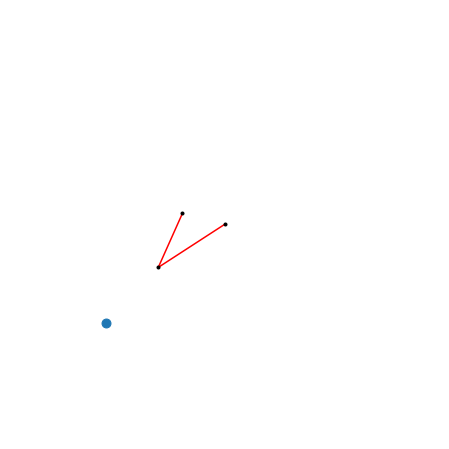In :
robot = Robot([4, 3])
robot.set_goal([4, 5])
robot.animate(100, show_all_steps=True)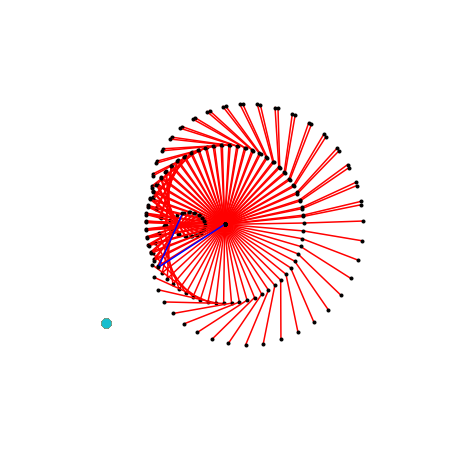# Reinforcement Learning Problem for Controlling the Robot¶

To define the reinforcement learning problem for controlling this robot, and trying to move the gripper as close to the goal as you can, we need to define the three main functions that define a reinforcement learning problem. These are pretty easy with the functions available to us in the Robot class. We will also need a function to represent the joint angles as sines and cosines.

In :
def angles_to_sin_cos(angles):
return np.hstack((np.sin(angles), np.cos(angles)))

def initial_state(robot):
robot.update_joints(np.random.uniform(-2 * pi, 2 * pi, size=(robot.n_links)))
angles = robot.get_angles()
state = angles_to_sin_cos(angles)
return state

def next_state(robot, action):
angles = robot.get_angles()
state = angles_to_sin_cos(angles)
return state

def reinforcement(robot):
'''Objective is to move gripper to the goal location as quickly as possible.'''
dist_to_goal = robot.dist_to_goal()
return dist_to_goal

In :
angles_to_sin_cos([0, pi/4, pi/2, pi, -pi])

Out:
array([ 0.00000000e+00,  7.07106781e-01,  1.00000000e+00,  1.22464680e-16,
-1.22464680e-16,  1.00000000e+00,  7.07106781e-01,  6.12323400e-17,
-1.00000000e+00, -1.00000000e+00])
In :
initial_state(robot)

Out:
array([-0.16659304, -0.2447326 , -0.98602574, -0.96959061])
In :
next_state(robot, [-1, 1])

Out:
array([ 0.73970145, -0.94811195, -0.67293519, -0.31793666])
In :
reinforcement(robot)

Out:
10.985973763956626

Now we need our two workhorse functions, epsilon_greedy and make_samples.

In :
def epsilon_greedy(robot, Qnet, valid_actions, epsilon):
state = angles_to_sin_cos(robot.get_angles())

if np.random.uniform() < epsilon:

# Random Move
actioni = np.random.randint(valid_actions.shape)
action = valid_actions[actioni]

else:
# Greedy Move

Qs = [Qnet.use(np.hstack((state, a)).reshape((1, -1))) for a in valid_actions]
ai = np.argmin(Qs)
action = valid_actions[ai]

Q = Qnet.use(np.hstack((state, action)).reshape((1, -1)))

return action, Q

In :
def make_samples(robot, Qnet, initial_state_f, next_state_f, reinforcement_f,
valid_actions, n_samples, epsilon):

X = np.zeros((n_samples, Qnet.n_inputs))
R = np.zeros((n_samples, 1))
Qn = np.zeros((n_samples, 1))

state = initial_state_f(robot)
state = next_state_f(robot,  * robot.n_links)  # 0 action for all joints
action, _ = epsilon_greedy(robot, Qnet, valid_actions, epsilon)

# Collect data from numSamples steps
for step in range(n_samples):

next_state = next_state_f(robot, action)
r = reinforcement_f(robot)
next_action, next_Q = epsilon_greedy(robot, Qnet, valid_actions, epsilon)

X[step, :] = np.hstack((state, action))
R[step, 0] = r
Qn[step, 0] = next_Q

state, action = next_state, next_action

return (X, R, Qn)


# Reinforcement Learning Training Algorithm¶

In :
def setup_standardization(Qnet, Xmeans, Xstds, Tmeans, Tstds):
Qnet.stand_params = {}
Qnet.stand_params['Xmeans'] = np.array(Xmeans)
Qnet.stand_params['Xstds'] = np.array(Xstds)
Qnet.stand_params['Tmeans'] = np.array(Tmeans)
Qnet.stand_params['Tstds'] = np.array(Tstds)

def train(robot, n_hiddens_list, valid_actions, n_trials, n_steps_per_trial, n_scg_iterations,
final_epsilon, gamma=0.8):

epsilon_decay = np.exp(np.log(final_epsilon) / (n_trials))  # to produce this final value

Qnet = nn.NeuralNetwork(n_inputs, n_hiddens_list, 1)

means_state =  * robot.n_links * 2
stds_states = [0.5] * robot.n_links * 2
setup_standardization(Qnet, means_state + means_actions, stds_states + stds_actions, , )

epsilon = 1         # initial epsilon value
epsilon_trace = []
r_mean_trace = []
r_trace = []
for trial in range(n_trials):

# Collect n_steps_per_trial samples

. . .

# Train Qnet on these samples for n_scg_iterations

. . .

# Update the three traces

. . .

# Decay epsilon

. . .

if trial + 1 == n_trials or (trial + 1) % (n_trials // 10) == 0:
print(f'Trial {trial+1}: Mean R {r_mean_trace[-1]:.2f}')

return Qnet, r_mean_trace, r_trace, epsilon_trace

In :
single_joint_actions = [-0.1, 0, 0.1]

In :
robot = Robot([3., 3.])
robot.set_goal([5., 6.])

In :
robot

Out:
<__main__.Robot at 0x7f64404b11c0>
In :
n_hiddens_list = [50, 10]
n_trials = 200
n_steps_per_trial = 1000
n_scg_iterations = 4
final_epsilon = 0.01
gamma = 0.9

np.random.seed(42)
Qnet, r_mean_trace, r_trace, epsilon_trace = train(robot, n_hiddens_list, valid_actions, n_trials,
n_steps_per_trial, n_scg_iterations,
final_epsilon, gamma)

Trial 20: Mean R 8.55
Trial 40: Mean R 7.17
Trial 60: Mean R 7.29
Trial 80: Mean R 7.13
Trial 100: Mean R 6.80
Trial 120: Mean R 3.12
Trial 140: Mean R 1.58
Trial 160: Mean R 1.57
Trial 180: Mean R 1.84
Trial 200: Mean R 1.10

In :
import matplotlib.pyplot as plt

plt.figure(figsize=(10, 10))
plt.clf()
plt.subplot(3, 1, 1)
plt.plot(r_mean_trace)
plt.xlabel('Trial')
plt.ylabel('Mean R per Trial')

plt.subplot(3, 1, 2)
plt.plot(r_trace)
plt.xlabel('Step')
plt.ylabel('Value of $r_t$')

plt.subplot(3, 1, 3)
plt.plot(epsilon_trace)
plt.xlabel('Trial')
plt.ylabel('Epsilon');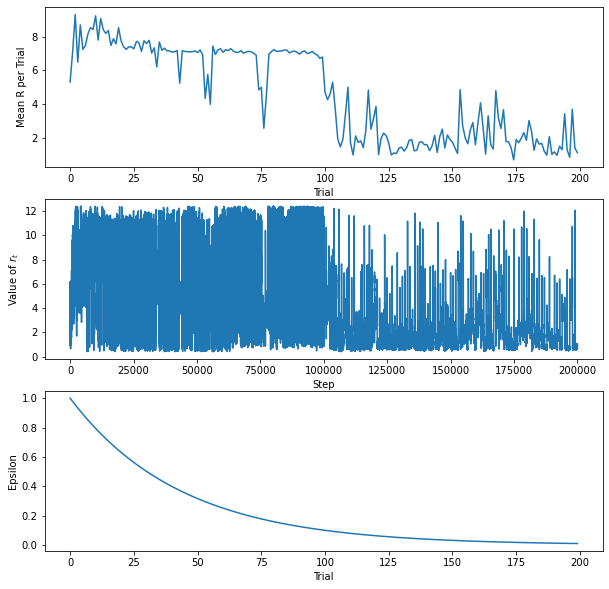In :
import numpy as np
np.random.seed(1234)
initial_state(robot)
robot.animate(100, Qnet)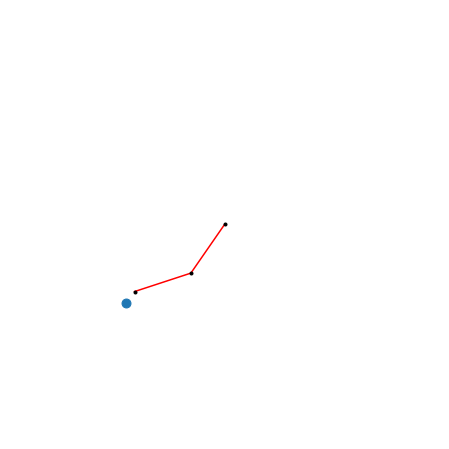In :
np.random.seed(1234)
initial_state(robot)
robot.animate(100, Qnet, show_all_steps=True)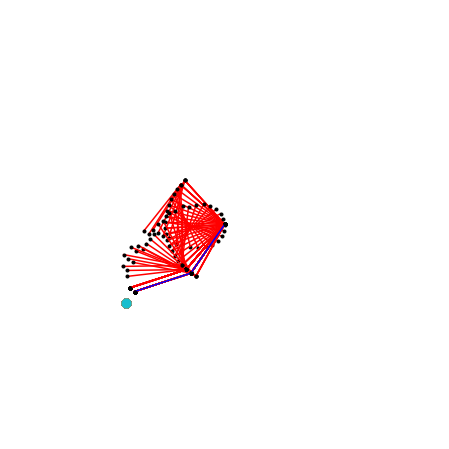In :
np.random.seed(4444)
initial_state(robot)
robot.animate(100, Qnet, show_all_steps=True)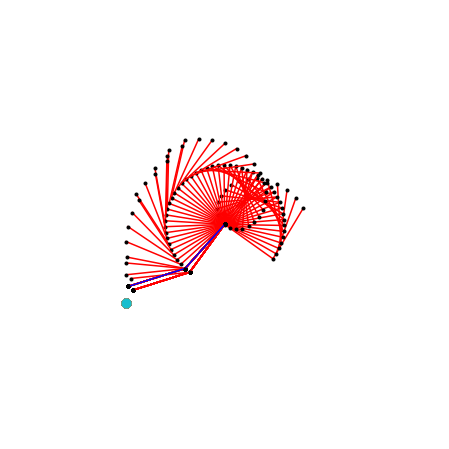# Required Experiments¶

Show the results and discuss what you see. Which parameter values result in the best performance during and at the end of training?

## Good parameter values¶

Find values for parameters n_hiddens_list, n_trials, n_steps_per_trial, n_scg_iterations, and gamma that result in Mean R per Trial plot that shows fairly steady decrease to value close to 0.

Again, find good values for the parameters.

## Increase number of valid actions¶

We have been using just three different actions for each joint, [-0.1, 0, 0.1]. Allow more precision in the control by changing this list of valid actions for each joint to [-0.1, -0.05, 0, 0.05, 0.1].

As before, find good values for the parameters.

# Extra Credit¶

As discussed in class for the marble control, make modifications to allow a variable goal. Add the goal coordinates to the state input to the Qnet. Demonstrate success by plotting r_mean_trace for four different, fixed, goals.